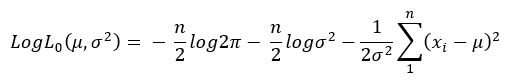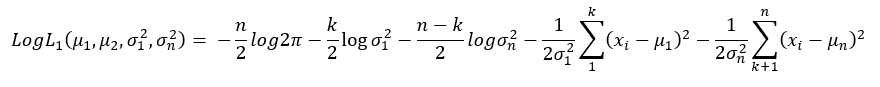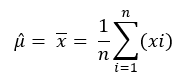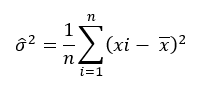# 1.1 - 8.10 - ChangePointDetection (ML Engine) - Teradata Vantage

## Teradata Vantage™ - Machine Learning Engine Analytic Function Reference

Product
Release Number
1.1
8.10
Published
October 2019
Content Type
Programming Reference
Publication ID
B700-4003-079K
Language
English (United States)

The change-point search method for retrospective change-point detection, binary segmentation, uses this procedure:

1. Search the data for the first change point.
2. At that change point, split the data into two parts.
3. In each part, select the change point with the minimum loss.
4. Repeat this procedure until there are either no new change points or the maximum number of change points.

Binary segmentation is an approximation method, because the change point is decided with only part of the data. However, this method is efficient and has an O(n log n) computational cost, where n is the number of data points.Taking normal distribution as an example, the change-point problem is to test the following null hypothesis:

H 0:μ = μ 1 = μ 2 = … = μ n and σ 2 = σ 1 2 = σ 2 2 = … σ n 2

as opposed to the alternatives,

H 1:μ 1 = … = μ k 1 μ k 1+1 = … μ k 2 ≠ ... ≠ μ k q+1 = ...= μ n

and

σ 1 2 = … = σ k 1 2σ k 1+1 2 = … = σ k 2 2 ≠ ... ≠ σ k q+1 2 = … = σ n 2

Binary segmentation performs the following tests in each iteration:

H 1:μ 1 = … = μ k 1 μ k 1+1 = … = μ n

and

σ 1 2 = … = σ k 1 2σ k 1+1 2 = … σ n 2

These are the formulas for the log likelihood functions H 0 and H 1:These are the formulas for the maximum likelihood estimation of μ and σ2:From the preceding formulas, the binary segmentation algorithm computes max LogL 1 by giving k different values. Then, to check for a change point, the algorithm compares the difference between max LogL 1 and LogL 0 to the penalty value.

If the algorithm detects a change point, it adds that change point to its list of candidate change points and splits the data into two parts. From the candidate change points that the algorithm finds in the two parts, it selects the one with the minimum loss.

The ChangePointDetection function detects change points in a stochastic process or time series, using retrospective change-point detection, implemented with these algorithms:

• Search algorithm: binary search
• Segmentation algorithm: normal distribution and linear regression

Use this function when the input data can be stored in memory and the application does not require a real-time response. If the input data cannot be stored in memory, or the application requires a real-time response, use the function ChangePointDetectionRT (ML Engine).